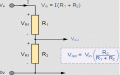# Need Help with Voltage circuit

#### JessyM

Joined Oct 11, 2019
10
Hello everyone, I'm not here often but when I need help this place is awesome, you guys are incredible.
Anyhow, I need two voltages from +5V source but one is variable. I need to switch(spdt) from +5V down to ~200mv, so I figure with a voltage divider, 2k2 and 85ohms should give me about 200mv. Next from the +5V, I need to go to 1.21V down to ~300mv with a pot, I can get that 1.21V from another voltage divider 5k6 and 1k8, but how can I vary down to ~300mv ?? I'm stumped, any help much appreciated, thank you in advance.

#### dl324

Joined Mar 30, 2015
14,318
What is the load on the voltage dividers?

#### LowQCab

Joined Nov 6, 2012
1,927
How much Current is required for each of these 2 mystery Loads.

Loads other than what the 5V supply is already providing power for ??

Or, at least in general, what sort of supply is creating the 5V ?
and how clean and stable must the 2 Voltages be ?
.
.
.

#### JessyM

Joined Oct 11, 2019
10
Sorry for delay, I don't know much about this circuit myself trying to learn at the same time as modifying it. looks like the 5V coming from 8255 IC and triggers 4066 switches to select either pulse wave or sine wave except the sine wave is going into an op amp then going to other places on this circuit board which is part of a bigger system., so basically just wanting to control it by a spdt and a pot, not sure I would have those resistor values to try. thank you for the help.

#### dl324

Joined Mar 30, 2015
14,318
I don't know much about this circuit myself trying to learn at the same time as modifying it. looks like the 5V coming from 8255 IC and triggers 4066 switches to select either pulse wave or sine wave except the sine wave is going into an op amp then going to other places on this circuit board which is part of a bigger system
Instead of trying to describe the circuit with words, post a schematic so we can see with a glance what you're talking about.

#### MrChips

Joined Oct 2, 2009
25,928
Sorry for delay, I don't know much about this circuit myself trying to learn at the same time as modifying it. looks like the 5V coming from 8255 IC and triggers 4066 switches to select either pulse wave or sine wave except the sine wave is going into an op amp then going to other places on this circuit board which is part of a bigger system., so basically just wanting to control it by a spdt and a pot, not sure I would have those resistor values to try. thank you for the help.
You would think that this is easy with a voltage divider. Well it isn't.

Show us exactly what you are trying to do. Pictures and photos are much better than words. We need to see the whole picture, figuratively and in reality.

#### MrChips

Joined Oct 2, 2009
25,928
Voltage Divider Design

Here is your basic voltage divider:
ΩVout = Vin x R2 / (R1 +R2)

Let us take an example.
Suppose Vin = 10V and we wish Vout = 1V

We make R1 = 9 x R2

Here are three possible solutions (using extreme values as examples)
1) R1 = 9Ω, R2 = 1Ω

2) R1 = 9kΩ, R2 = 1kΩ

3) R1 = 9MΩ, R2 = 1MΩ

All three will satisfy the requirement that Vout = 1V
Which one is the right or preferred solution?

With inspection one realizes that there must be an infinite number of solutions.
Which one do we choose?

This is where we need to know more about current.
How much current can Vin supply?
How much current will be drawn from Vout?

As soon as you draw current from Vout, the value of Vout will fall.
The Voltage Divider formula only works if zero current is taken from Vout.

In electronics (and other engineering fields) we oftentimes resort to the "it's good enough" solution.
The rule of thumb is, don't take more than one-tenth of what is being supplied. And the inverse applies.

For example, if you need 1mA from Vout, make sure that you have more than 10mA flowing in R1.
Using this criteria, we would select
R1 = 900Ω, R2 = 100Ω

As an exercise, determine the new value of Vout when a 1kΩ load is applied across R2.

Now if you need exactly 1mA @ 1V into the load, you will have to go back and recalculate your values,
because now you have imposed a 1kΩ load into the circuit.
Determine the new value of R2 in order to satisfy the above requirement.